# SUM IF using lookup values in excel

In this article, we will learn to SUM IF using lookup values in Excel.

In simple words, while working with different data tables, sometimes we need to calculate values in first table based values in another table. Condition is to get the sum of values in the first table using the second table values

For this article we will be needing the use the following functions:

SUMPRODUCT function is a mathematical function in Excel. It operates on multiple ranges. It multiplies the corresponding arrays and then adds them.

Total= ( A1 * B1 * C1 * .. + A2 * B2 * C2 * ..  + …)

Syntax:

= SUMPRODUCT ( array1 , [array2] , ...)

array : It is the range or a list of values.

SUMIF function in Excel returns the SUM of the numbers having fulfilled the specified criteria. SUM range & criteria range can be same or different.

Generic Excel SUMIF Formula:

= SUMIF ( criteria_range , criteria , sum_range)

criteria_range : range where condition is applied.
criteria: condition to apply on criteria_range.
sum_range : sum range on the basis of criteria.

Now we will make a formula out of these functions. Here we will given the data and we needed sum results where value matches the value in lookup table.
Generic formula:

= SUMPRODUCTSUMIF ( result, records, sum_nums))

result : values to be matched in lookup table

record : values in the first table

sum_nums : values to sum as per matching values.

Let's test this formula via running it on an example

Here we are calculating the total points of a football playing team using the points table or lookup table.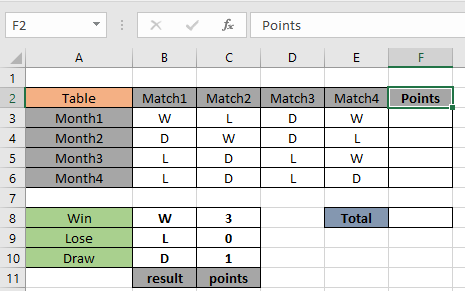Here result ( B8:B10 ) given as named range & points ( C8:C10 ) given as named range.

Now we will use the below formula to get the SUM of points for the Month1 in F3 cell
Use the Formula:

= SUMPRODUCTSUMIF ( result, B3:E3, points))

Explanation:

• SUMIF function matches the result with values in B3:E3.
• Then points corresponding to the result are returned to the SUMPRODUCT function.
• SUMPRODUCT function returns the SUM of values given in as array to the function.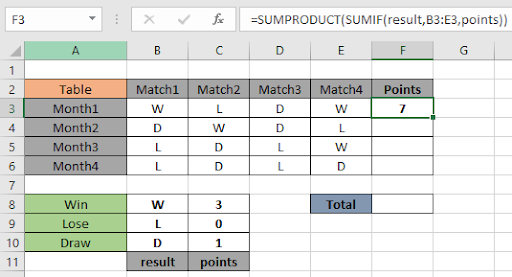As you can see here the total points scored by the team in First month is 7.

3 (W) + 0 (L) + 1 (D) + 3 (W) = 7 points

Copy the formula in other cells, select the cells taking the first cell where the formula is already applied, use shortcut key Ctrl + D or using the drag down cell option in excel.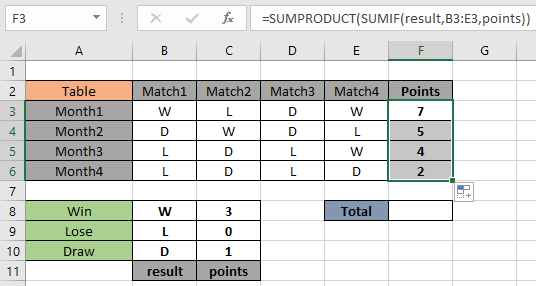As you can see in the above snapshot the points scored in the 4 different months.

Get the SUM of all points for 4 months.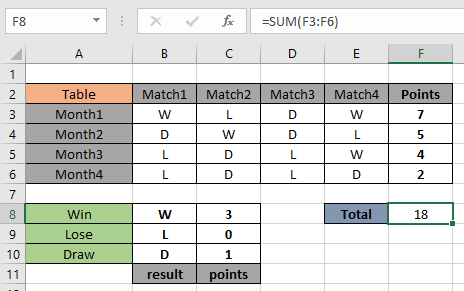That means the team scored a total of 18 points in 4 months.

Alternative method
Use the SUM and array method in place of using the SUMPRODUCT function.
Use the formula:

{ = SUM ( SUMIF ( result , B3:E3 , points )) }

Curly braces is the array method of suggesting the function calculate all the returned value by SUMIF function.

Note : Do not use the curly braces symbols manually. Use the Ctrl + Shift + Enter to use the array method in Excel.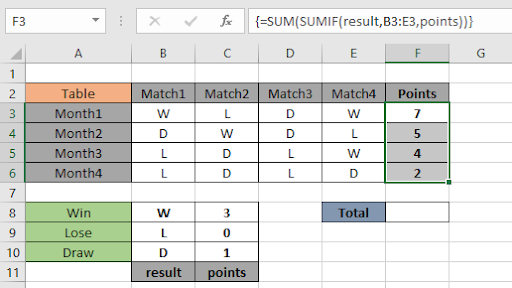As you can see from the above formula the you can get total points using conditional lookup table.

Notes:

1. The formula only works with numbers.
2. The formula works only when there are no duplicates in the lookup table
3. The SUMPRODUCT function considers non - numeric values as 0s.
4. The SUMPRODUCT function considers logic value TRUE as 1 and False as 0.
5. The argument array must be of same length else the function

Hope this article about how to Return SUM only from formulas in Excel is explanatory. Find more articles on SUMPRODUCT functions here. Please share your query below in the comment box. We will assist you.

Related Articles

How to use the SUMPRODUCT function in Excel: Returns the SUM after multiplication of values in multiple arrays in excel.

SUM if date is between : Returns the SUM of values between given dates or period in excel.

Sum if date is greater than given date: Returns the SUM of values after the given date or period in excel.

2 Ways to Sum by Month in Excel: Returns the SUM of values within a given specific month in excel.

How to Sum Multiple Columns with Condition: Returns the SUM of values across multiple columns having condition in excel

Popular Articles

50 Excel Shortcut to Increase Your Productivity

Edit a dropdown list

Absolute reference in Excel

If with conditional formatting

If with wildcards

Vlookup by date

Convert Inches To Feet and Inches in Excel 2016

Join first and last name in excel

Count cells which match either A or B

Terms and Conditions of use

The applications/code on this site are distributed as is and without warranties or liability. In no event shall the owner of the copyrights, or the authors of the applications/code be liable for any loss of profit, any problems or any damage resulting from the use or evaluation of the applications/code.